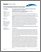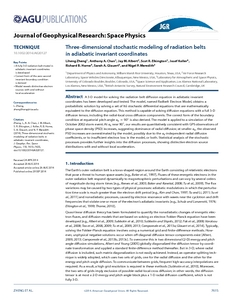nerc.ac.uk

Zheng, Liheng; Chan, Anthony A.; Albert, Jay M.; Elkington, Scot R.; Koller, Josef; ; ; . 2014 Three-dimensional stochastic modeling of radiation belts in adiabatic invariant coordinates. Journal of Geophysical Research: Space Physics, 119 (9). 7615-7635. https://doi.org/10.1002/2014JA020127Preview
Text
jgra51292.pdf - Published Version

Abstract/Summary

A 3-D model for solving the radiation belt diffusion equation in adiabatic invariant coordinates has been developed and tested. The model, named REM (for Radbelt Electron Model), obtains a probabilistic solution by solving a set of Itô stochastic differential equations that are mathematically equivalent to the diffusion equation. This method is capable of solving diffusion equations with a full 3-D diffusion tensor, including the radial-local cross diffusion components. The correct form of the boundary condition at equatorial pitch-angle α0 = 90° is also derived. The model is applied to a simulation of the October 2002 storm event. At α0 near 90°, our results are quantitatively consistent with GPS observations of phase-space density (PSD) increases, suggesting dominance of radial diffusion; at smaller α0, the observed PSD increases are overestimated by the model, possibly due to the α0-independent radial diffusion coefficients, or to insufficientelectron loss in the model, or both. Statistical analysis of the stochastic processes provides further insights into the diffusion processes, showing distinctive electron source distributions with and without local acceleration.

Item Type: Publication - Article https://doi.org/10.1002/2014JA020127 BAS Programmes > Polar Science for Planet Earth (2009 - ) > Climate 21699380 radiation belt, diffusion equation, fully 3-D model, adiabatic invariant coordinates, stochastic differential equation 01 Sep 2014 08:41 +0 (UTC) http://nora.nerc.ac.uk/id/eprint/508279View Item